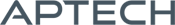# Maximum Likelihood MT

Maximum Likelihood MT

Availability: Out of stock

Maxlikmt provides a set of procedures to estimate the parameters of models by the maximum likelihood method and perform statistical inference.
Overview

## Maximum Likelihood MT

MaxlikMT provides a suite of flexible, efficient and trusted tools for the solution of the maximum likelihood problem with bounds on the parameters.

### Version 3.0 is easier to use than ever!

• New syntax options eliminate the need for PV and DS structures:
• Decreasing the required code up to 25%.
• Decreasing runtime up to 20%.
• Simplifying usage.
• Optional dynamic arguments make it simple and transparent to add extra data arguments beyond model parameters to your objective function.
• Updated documentation and examples.
• Fully backwards compatible with MLMT 1.0-2.0

### Features include:

• A variety of descent and line search algorithms.
• Computation of analytical and numerical derivatives.
• Dynamic algorithm switching.
• Multiple methods for statistical inference.

Default selections allow you to see results quickly with minimum programming effort, while an array of modelling parameters allows the flexibility to solve custom problems.

Platform: Windows, Mac and Linux.

Requirements: GAUSS/GAUSS Light version 17 or higher.

Features

## Key Features

### Statistical Inference

Maxlikmt provides methods for statistical inference of weighted or unweighted, bounded or unbounded maximum likelihood models.

• Inverted Hessian covariance matrix
• Heteroskedastic-consistent covariance matrix
• Wald confidence limits
• Likelihood ratio statistics
• Bootstrap
• Likelihood profile and Profile ‘t’ traces

### Descent methods

• BFGS (Broyden, Fletcher, Goldfarb, and Powell)
• DFP (Davidon, Fletcher, and Powell)
• Newton
• BHHH (Berndt, Hall, Hall, and Hausman)

### Line search methods

• STEPBT
• Brent’s method
• HALF
• Strong Wolfe’s Conditions

### Flexible

• Bounded parameters.
• Specify fixed and free parameters.
• Dynamic algorithm switching.
• Compute all, a subset, or none of the derivatives numerically.
• Easily pass data other than the model parameters as extra input arguments. New!
• Methods to simply create matrices needed for log-likelihood computation from subsets of the parameters.

### Efficient

• Option to avoid computations that are the same for the log-likelihood function and derivatives.
• The tremendous speed of user-defined procedures in GAUSS speeds up your estimation.

### Trusted

For more than 30 years, leading researchers have trusted the efficient and numerically sound code in the GAUSS maximum likelihood estimation tools to keep them at the forefront of their fields.

## Details

Novice users will typically leave most of these options at the default values. However, they can be a great help when tackling more difficult problems.

Control options
Parameter bounds Simple parameter bounds of the type: lower_bd ≤ x_i ≤ upper_bd
Descent algorithms BFGS, DFP, Newton and BHHH.
Algorithm switching Specify descent algorithms to switch between based upon the number of elapsed iterations, a minimum change in the objective function or line search step size.
Weights Observation weights.
Covariance matrix type Compute a ML covariance matrix, a QML covariance matrix, or none.
Alpha Probability level for statistical tests.
Line search method STEPBT (quadratic and cubic curve fit), Brent’s method, BHHHStep, half-step or Strong Wolfe’s Conditions.
Active parameters Control which parameters are active (to be estimated) and which should be fixed to their start value.
Gradient Method Either compute an analytical gradient, or have Maxlikmt compute a numerical gradient using the forward, central or backwards difference method.
Hessian Method Either compute an analytical Hessian, or have Maxlikmt compute a numerical Hessian using the forward, central or backwards difference method.
Gradient check Compares the analytical gradient computed by the user supplied function with the numerical gradient to check the analytical gradient for correctness.
Random seed Starting seed value used by the random line search method to allow for repeatable code.
Print output Controls whether (or how often) iteration output is printed and whether a final report is printed.
Gradient step Advanced feature: Controls the increment size for computing the step size for numerical first and second derivatives.
Random search radius The radius of the random search if attempted.
Maximum iterations Maximum iterations to converge.
Maximum elapsed time Maximum number of minutes to converge.
Maximum random search attempts Maximum allowed number of random line search attempts.
Convergence tolerance Convergence is achieved when the direction vector changes less than this amount.

Product Inquiry

3 + 1 = enter the result ex:(3 + 2 = 5)

﻿

### Try GAUSS for 14 days for FREE

See what GAUSS can do for your data